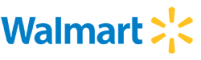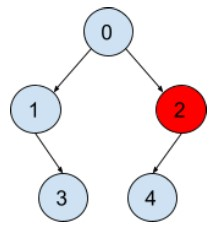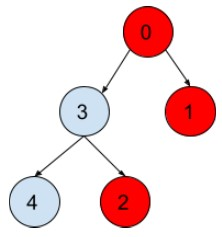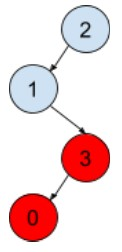New update is available. Click here to update.

# Locked Binary Tree

Contributed by
Rakesh Tiwari
Easy0/40
8 mins
100 %2 upvotes## Problem Statement

#### A node will be locked only when some or all of the ancestors or the node itself is locked.

##### EXAMPLE:
``````Input:
'N' = 5, ‘K’ = 3
‘ARR’ = [-1, 0, 0, 1, 2]
‘LOCK’ = [0, 0, 1, 0, 0]

Output: ‘1’
````````````In the above tree in the simple path from node ‘4’ to root ‘1,’ the nodes encountered are [0, 1, 3], and no node from the set is locked. Hence node ‘3’ can be locked.
``````
Detailed explanation ( Input/output format, Notes, Images )
##### Constraints :
``````1 <= 'T' <= 10
1 <= 'N' <= 10^5
0 <= ‘K’ <= ‘N-1’
0 <= ‘PAR[i]’ <= ‘N-1’
0 <= ‘LOCK[i]’ <= 1

Time Limit: 1 sec
``````
##### Sample Input 1 :
``````2
5 0
-1 0 3 0 3
1 1 1 0 0
4 1
3 2 -1 1
1 0 0 1
``````
##### Sample Output 1 :
``````0
1
``````
##### Explanation Of Sample Input 1 :
``````In the first test case,
````````````In the above tree the target node ‘0’ is itself locked, so it cannot be locked.

In the second test case,
````````````In the above tree in the simple path from node ‘1’ to root ‘2’ the nodes encountered are [1, 2], and no node from the set is locked.
``````
##### Sample Input 2 :
``````2
4 2
-1 0 1 1
0 1 0 0
4 3
-1 0 1 2
1 0 0 0
``````
##### Sample Output 2 :
``````0
0
``````AutocompleteConsole